# limit

(redirected from assimilation limit)
Also found in: Dictionary, Thesaurus, Medical, Financial.

## limit

limit, in mathematics, value approached by a sequence or a function as the index or independent variable approaches some value, possibly infinity. For example, the terms of the sequence 1-2, 1-4, 1-8, 1-16, … are obviously getting smaller and smaller; since, if enough terms are taken, one can make the last term as small, i.e., as close to zero, as one pleases, the limit of this sequence is said to be zero. Similarly, the sequence 3, 5, 31-2, 41-2, 33-4, 41-4, 37-8, 41-8, … is seen to approach 4 as a limit. However, the sequences 1, 2, 4, 8, 16, … and 1, 2, 1, 2, 1, 2, … do not have limits. Frequently a sequence is denoted by giving an expression for the nth term, sn; e.g., the first example is denoted by sn = 1-2n. The limit, s, of a sequence can then be expressed as lim sn = s, or in the case of the example, lim 1-2n = 0 (read “the limit of 1-2n as n approaches infinity is zero”). A sequence is a special case of a function. In many functions commonly encountered, the values of the independent variable (the domain) and those of the dependent variable (the range) may be any numbers, while for a sequence the domain is restricted to the positive integers, 1, 2, 3, … . The function y = 1-2x resembles the sequence used as an example, but note that x can take on values other than 1, 2, 3, … ; thus we find not only lim 1-2x = 0 but also lim 1-2x = 4. A more precise definition of the limit of a function is: The function y = f(x) approaches a limit L as x approaches some number a if, for any positive number ε, there is a positive number δ such that |f(x) − L| < ε if 0 < |x − a| < δ. Similarly, f(x) has the limit L as x becomes infinite if for any positive ε there is a δ such that |f(x) − L| < ε if |x| > δ.
The following article is from The Great Soviet Encyclopedia (1979). It might be outdated or ideologically biased.

## Limit

one of the basic concepts of mathematics. The limit is a constant that is approached without bound by a certain variable that depends on another variable when the latter changes in a specific way. The simplest form of the concept of limit is that of the limit of a sequence of numbers, by means of which we can define the concepts of limit of a function, limit of a sequence of points in space, and limit of so-called Riemann sums.

Limit of a sequence. Let there be given a sequence of real numbers xn, n = 1, 2, …. The number a is called the limit of this sequence if for any number ∊ > 0 there exists a number n such that for all numbers n ≥ n, the inequality ǀxnaǀ < ∊ is satisfied. In this case, one writes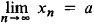(“lim” is the first three letters of the Latin limes).

xna as n → ∞

If a sequence has a limit, then it is said to converge. Thus, the sequence 1/n, n = 1, 2, …, converges and has as its limit the number 0. Not every sequence has a limit; for example, the sequence 1, –1, 1, …, (—1)n+1, … does not have a limit. A sequence that does not have a limit is called divergent. In geometrical terms, the existence of a limit a for a sequence means that every neighborhood of the point a contains all but (possibly) a finite number of the terms of the given sequence.

The following formulas hold for limits of sequences: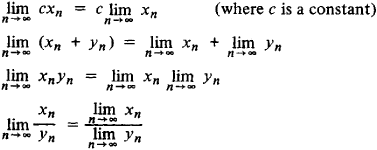These formulas hold under the assumption that the limits appearing in their right-hand sides exist; moreover, in the formula for the limit of the quotient xn/yn it is also necessary to require that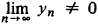If xnyn and the sequences xn and yn, n = 1, 2, …, converge, then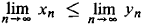that is, nonstrict inequalities are preserved by the limiting process: however, it does not follow from xn < yn that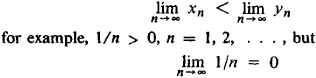If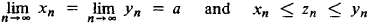then the sequence zn, n = 1, 2, …, converges to the same limit: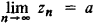A sequence αn, n = 1, 2, …, which converges to zero is called a null sequence. A sequence converges to some number if and only if the difference between the terms of the sequence and this number is a null sequence; in this way, the general concept of limit of a sequence reduces to the concept of a null sequence. Thus, for example, the sequence 1/2, 2/3, 3/4, …, n/(n + 1) has 1 as its limit, since the difference 1—n/(n + 1) = 1/(n + 1), n = 1, 2, …, is a null sequence.

Every nondecreasing (nonincreasing) sequence that is bounded above (below) converges. For example, if for a given number a we denote by an the approximate value of its kth root(where k is a natural number), which has n digits after the decimal point and is less than or equal to the root, then an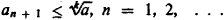and therefore the sequence an converges; moreover, from the inequality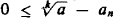≤ 10n it follows thatAnother example of a sequence that is nondecreasing (in fact, increasing) and bounded above is the sequence of perimeters of regular polygons inscribed in a circle, which converges to the circle’s circumference.

In order for an arbitrary sequence xn to converge, it is necessary and sufficient that it satisfy Cauchy’s criterion: for any number ∊ > 0, there exists a number N such that for all numbers mN and n ≥ N∊, ǀ xnxm ǀ ∊.

If xn, n = 1, 2, …, is a sequence such that for any number ∊ > 0 there exists a number n with the property that xn > ∊ (xn < —∊) for all nn, then we say that the sequence converges to infinity (minus infinity) and write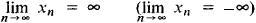These limits are said to be infinite. Sequences for whichare also of interest. For example,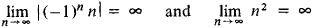It is obvious, of course, that sequences that converge to infinity or minus infinity are not convergent in the sense of the definition of convergence given earlier. In general, properties of finite limits do not carry over to infinite limits. For example, the sequences xn= n and yn = sin (nπ/2) — n converge to infinity and minus infinity, respectively, but the sequence xn + yn, n = 1, 2, …, is bounded and divergent.

Limit superior and limit inferior. From every bounded sequence we can select a convergent subsequence (the BolzanoWeierstrass theorem), and from every unbounded sequence we can select a subsequence that converges to infinity or minus infinity. Consider the (finite or infinite) limits of all convergent subsequences of a sequence xn, n = 1, 2, …. The largest of these limits is called the limit superior of xn and is denoted byand the smallest is called the limit inferior of xn and is denoted byFor example,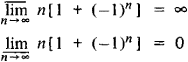A sequence has a finite or infinite limit if and only if its limit superior coincides with its limit inferior, in which case their common value is the limit of the sequence. If finite, the limit superior of a sequence may also be defined as that number a such that for any ∊ > 0 there are infinitely many terms of the sequence that are larger than a — ∊, and no more than a finite number larger than a + ∊.

Limit of a function. Let a real-valued function f be defined in some neighborhood of x0 that does not possibly contain x0. Such a neighborhood is called a deleted neighborhood of x0. The function f has a limit at x0 if for any sequence of points xn, n = 1, 2, …, xnx0, that approach x0, the sequence of functional values f(xn) converges to a number A, called the limit of the function f at x0 (or as xx0). In symbols,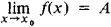or

f(x) → A as xx0

As a consequence of this definition, the properties of limits of a sum, product, and quotient of sequences can be carried over to limits of functions, as can the preservation of inequalities by the limiting process.

The definition of limit of a function may also be formulated without recourse to the concept of limit of a sequence: the number A is called the limit of the function f at the point x0 if for any number ∊ > 0 there exists a number δ > 0 such that for all points xx0 satisfying the conditions ǀxx0 ǀ < δ, xx0, the inequality ǀf(x)Aǀ < ∊ holds.

All fundamental elementary functions—constants, the power function xa, the exponential function ax, the trigonometric functions sin x, cos x, tan x, and cot x, and the inverse trigonometric functions arc sin x, arc cos x, arc tan x, and arc cot x—have limits at all interior points of their domain that coincide with their values at these points. But this is not true for all functions. Thus the functionwhich is the sum of an infinite geometric progression with ratio q = 1/(1 + x2), 0 < q < 1, has limit 1 at x = 0 (since f(x) = 1 + x2 for x ≠ 0), and this limit does not coincide with the value f(0) = 0 of f at zero. On the other hand, the function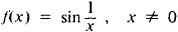does not have any limit as x0, since for the values xn = 1/(π/2 + ) the sequence of corresponding function values f(xn) = (—1)n does not have a limit.

In order for a function f to have a limit A as xx0, it is necessary and sufficient that f(x) = A + α(x), where α(x) → 0 as x → x0.

If in the determination of the limit of a function f at a point x0 only points x lying to the left (right) of x0 are considered, then the limit obtained is called the left-hand limit (right-hand limit) and is denoted by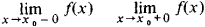A function has a limit at a point if its left-hand limit at that point equals its right-hand limit. The concept of limit of a function can be generalized even to the case when the argument tends to infinity.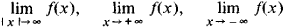For example,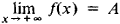means that for any ∊ > 0 there exists a δ > 0 such that for all x satisfying the condition x > δ, the inequality

ǀf(x)Aǀ < ∊

holds.

Examples of functions that always have limits are provided by monotone functions. Thus, if the function f is defined on the interval (a, b) and is nondecreasing, then at each point x, a < x < b, it has a finite left-hand and right-hand limit. The right-hand limit of f at a is finite if and only if f is bounded from below, and its left-hand limit at b is finite if and only if f is bounded from above. In general, a function may have a limit at a point without being monotone in any neighborhood of that point. For example, the function f(x) = x sin (1/x) tends to zero as x0 and oscillates an infinite number of times in every (deleted) neighborhood of zero.

A so-called intrinsic criterion (Cauchy’s criterion) for the existence of a limit of a function at a point consists in the following: the function f has a limit at a point x0 if and only if for each ∊ > 0 there exists a δ > 0 such that for all points x′ and x″ fulfilling the conditions ǀx′x0ǀ < δ, ǀx″x0ǀ < δ, x′x0, x″x0, the inequality ǀf(x″)f(x′ǀ) < ∊ is satisfied.

For functions, just as for sequences, one defines the concepts of infinite limits. Examples are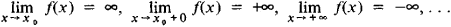In these cases, the function f is said to converge to infinity (or minus infinity) as xx0, xx0 + 0, or x → + ∞, respectively. For example,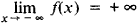means that for any ∊ > 0 there exists a δ > 0 such that for all x fulfilling the condition x < —δ, the inequality f(x) > ∊ is satisfied.

EXTENSION OF THE CONCEPT OF LIMIT OF A FUNCTION. If a function f is defined on a certain set E on the real line and the point x0 is such that any neighborhood of it contains points from the set E, we say that x0 is a limit point of E. In particular, ∞ is said to be a limit point of E if E contains a sequence that converges to ∞. We define the limit of a function f at x0 relative to E (briefly, but inaccurately, the limit of f relative to E) in much the same way as the earlier concept of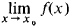except for the natural requirement that x belong to E:xE. In symbols,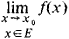If E is the set of natural numbers and we put f(n) = xn, n = 1, 2, …, then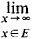reduces to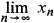that is, the earlier concept of limit of a sequence.

The function that is equal to 0 at rational x and 1 at irrational x does not have a limit as x → 0, whereas on the set of rational numbers it has the limit zero as x → 0. The concept of limit of a real-valued function at a limit point of a set can also be carried over to the case of a function of several variables. In this case, we may speak in particular of a limit in a given direction, along a given curve, along a given surface, and so forth. In addition, functions of several variables give rise to the concept of iterated limit, when the limit is taken successively with respect to different variables, for example,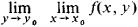The concept of limit can also be extended to functions that may assume complex, instead of just real, values.

Limit of Riemann sums. Still another important concept of limit arises in the definition of an integral. For example, let the function f be defined on the interval [a, b]. A set {xi} of points such that

a = x0 < x1 < … < xi, < … < xn-1 < xn = b

is called a partition of the interval [a, b]. Let xi-1 ≤ ξixi, Δxi = xixi-1, i = 1, 2, …, n. Then the sum f1x1 + f2) Δ x2 + … + fn) Δ xn is called a Riemann sum of the function f. The number A is the limit of the Riemann sums of f and is called the definite integral of f,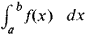if for any ∊ > 0 there exists a δ > 0 such that for any partition {xi} of the interval [a, b] for which Δxi < δ and any choice of the points ξb where xi-1 ≤ ξixi, i = 1, 2, …, n, the inequality

ǀf1) Δ x1 + f2) Δ x2 + … + fn) Δ xnAǀ ≤ ∊

is satisfied. The limit of Riemann sums may also be introduced by means of limits of sequences.

Generalizations. In view of the diversity of the special types of limits used in mathematics, the tendency naturally arose to include them as special cases of some general concept of limit. For example, one may introduce a concept of limit that generalizes both the concepts of limit of a function and limit of Riemann sums.

A set S of nonempty subsets of some set E is said to be a directed system if for every two subsets A and B of S either AB or BA, and the intersection of all the sets of S is empty. Let a real-valued function f be defined on E. The number a is called the limit of f with respect to S if for any ∊ > 0 there exists a set A in S such that the inequality ǀ f(x)aǀ < ∊ is satisfied at all points of A.

In the definition of limit of a function f at a point x0, the directed system may be taken to be the collection of all deleted neighborhoods of x0 with sufficiently small radii.

In the definition of the limit of Riemann sums of a function f given on an interval [a, b], it is necessary to consider the set E whose elements are all the possible partitions of the interval [a, b] together with choices of the ξi. The subsets Eη of the set E corresponding to partitions for which the lengths Δxi do not exceed η form a directed system. The limit of the Riemann sums (which are obviously functions defined on the set E) with respect to this directed system is the integral.

The concept of limit can be generalized to wider classes of functions, for example, functions defined on partially ordered sets or functions that map one space (metric or, more generally, topological) onto another. The problem of defining the concept of limit has been most fully solved in topology, and it means in the general case that some object, denoted by f(x), that varies with another object, denoted by x, can approach an object A as closely as desired, provided that the object x is sufficiently close to the object x0. Basic to these concepts of limit is the concept of closeness of the objects x and x0, f(x) and A, which need mathematical definition. Only after this has been done can a clear meaning be attached to the above definition of limit. Various ideas of closeness are studied in topology.

Limits of a different nature not connected with topology are also encountered, for example, the concept of limit of a sequence of sets. A sequence of sets An, n = 1, 2, …, is convergent if there exists a set A, called its limit, such that each of its elements belongs to all the sets An, beginning with a certain value of n, and all the elements in the union of all the sets An that do not belong to A belong to only a finite number of the An.

Historical survey. The ancient Greeks came close to developing the concept of limit in the calculation of areas and volumes of certain figures and solids by the method of exhaustion. Archimedes, in considering sequences of inscribed and circumscribed polygons (polyhedrons), showed by the method of exhaustion that the difference between their areas (volumes) could be made smaller than any positive quantity fixed in advance. The method of exhaustion, which was related to the idea of infinitesimals, was the germ of the theory of limits. However, the concept of limit in ancient Greek mathematics was not clearly formulated, and the foundations for a general theory of limits were not created.

A new stage in the development of the limit concept occurred with the creation of the differential and integral calculus. In their calculations of areas and volumes, Galileo, Kepler, Cavalieri, B. Pascal, and others widely used the method of “indivisibles,” the method of actual infinitesimals, that is, infinitesimals which, according to their ideas, were unchangeable quantities not equal to zero but nevertheless less in absolute magnitude than any positive finite quantities. The method of exhaustion also underwent changes and developments in this period (Gregory of Saint Vincent, P. Guldin, C. Huygens).

Attempts were also made to create a general theory of limits on the basis of an intuitive concept of limit. Newton devoted the first section of the first book (De motu corporum) of his Philosophiae naturalis principia mathematica to a form of the theory of limits under the name “method of prime and ultimate ratios,” which he took as the basis of his calculus of fluxions. In this theory, Newton proposed the replacement of actual infinitesimals by “potential” infinitesimals, which only in the process of changing become less in absolute magnitude than any positive finite quantity. Newton’s viewpoint was a substantial step forward in the development of the idea of limit. The concept of limit, which was taken up by mathematicians of the 17th century, was gradually analyzed and refined in the 18th century by Euler, d’Alembert, L. Carnot, and the Bernoulli brothers. In this period it was only used in attempts to explain the correctness of the differential and the integral calculus and had not yet become a method for working out problems in mathematical analysis.

The modern theory of limits arose at the beginning of the 19th century in connection with the study of the properties of different classes of functions, primarily continuous functions, as well as in connection with attempts to prove the existence of a number of basic objects of mathematical analysis (integrals of functions of real and complex variables, sums of series, solutions of algebraic as well as more general equations). The concept of limit first became the basis for the construction of mathematical analysis by A. Cauchy, who obtained basic conditions for the existence of the limit of a sequence and fundamental theorems on limits. Above all, Cauchy gave the criterion for convergence of a sequence, which now bears his name. Finally, he defined the integral as the limit of what are now called Cauchy or Riemann sums and studied its properties, starting from this definition.

The concept of limit of a sequence and of a function was ultimately formulated on the basis of the theory of real numbers in the works of B. Bolzano and K. Weierstrass. Further generalizations of the concept of limit were obtained by S. O. Shamunovskii (published 1923), the American mathematicians E. H. Moore and H. L. Smith (1922), and the French mathematician H. Cartan (1937).

### REFERENCES

Aleksandrov, P. S. Vvedenie v obshchuiu teoriiu mnozhestv i funktsii. Moscow-Leningrad, 1948.
Il’in, V. A., and E. G. Pozniak. Osnovy matematicheskogo analiza, 3rd ed., vols. 1–2. Moscow, 1971–73.
Kudriavtsev, L. D. Matematicheskii analiz, 2nd ed., vols. 1–2. Moscow, 1970.
Nikol’skii, S. M. Kurs matematicheskogo analiza, vols. 1–2. Moscow, 1973.
Smirnov, V. I. Kurs vysshei matematiki, 22nd ed., vol. 1. Moscow, 1967.

L. D. KUDRIAVTSEV

## limit

[′lim·ət]
(mathematics)
A function ƒ(x) has limit L as x tends to c if given any positive number ε (no matter how small) there is a positive number δ such that if x is in the domain of ƒ, x is not c, and | x-c | < δ,="" then="" |ƒ="">(x)-L | < ε;="" written="">
A sequence {an : n = 1, 2,…} has limit L if given a positive number ε (no matter how small), there is a positive integer N such that for all integers n greater than N, | an -L | <>
McGraw-Hill Dictionary of Scientific & Technical Terms, 6E, Copyright © 2003 by The McGraw-Hill Companies, Inc.

## limit

1. Maths
a. a value to which a function f(x) approaches as closely as desired as the independent variable approaches a specified value (x = a) or approaches infinity
b. a value to which a sequence an approaches arbitrarily close as n approaches infinity
c. the limit of a sequence of partial sums of a convergent infinite series
2. Maths one of the two specified values between which a definite integral is evaluated
Collins Discovery Encyclopedia, 1st edition © HarperCollins Publishers 2005
Site: Follow: Share:
Open / Close